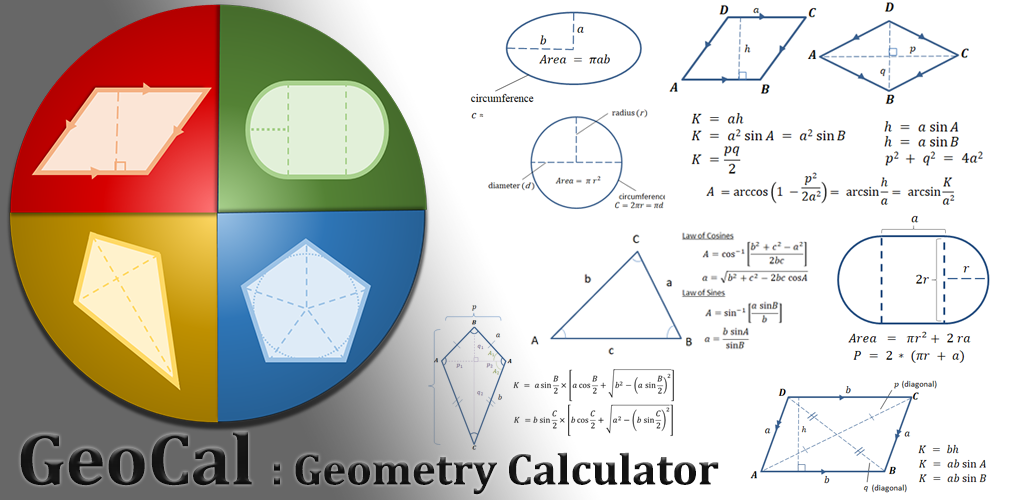Select Page

# GeoCal : Geometry CalculatorAvailable on Playstore , AppGallery

Currently support 2D shapes:

• Circle
• Ellipse (Oval)
• Triangle : Equilateral Triangle
• Triangle : Pythagorean
• Triangle : Area (basic formula)
• Triangle : Area by sides (Heron’s formula)
• Triangle : Angles and Sides (Trigonometry)